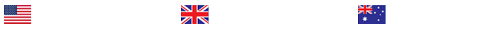Describe the assumptions of normality and homogeneity of variance.

Activity Description

You will submit one Word document, including your SPSS output.

1. Why do we care whether the assumptions required for statistical tests are met? (Tip: You might also want to write your answer on a note

card you paste to your computer.)

2. Open the data set that you corrected in Activity #1 for DownloadFestival.sav. You will use the following variables: Day1, Day2, and Day3 (hygiene variable for all three days). Create a simple histogram for each variable. Choose to display the normal curve (under Element Properties) and title your charts. Copy these plots into your Word document.

3. Now create probability-probability (p-p) plots for each variable. This output will give you additional information. Read over the Case Processing Summary. Notice that there is missing data for Days 2 and Day 3. Copy only the Normal p-p Plots into your Word document (you do not need to copy the beginning output nor the Detrended Normal p-p Plots).

4. Examining the histograms and p-p plots, describe the dataset with particular attention toward the assumption of normality. For each day, do you think the responses are reasonably normally distributed? (Just give your impression of the data.) Why or why not?

5. Using the same dataset and the Frequency command, calculate the standard descriptive measures (mean, median, mode, standard deviation, variance and range) as well as kurtosis and skew for all three hygiene variables. Paste your output into your Word document (you do not need to paste the Frequency Table). What does the output tell you? You will need to comment on: sample size, measures of central tendency and dispersion and well as kurtosis and skewness. You will need to either calculate z scores for skewness andkurtosis or use those given in the book to provide a complete answer. Bottom line: is the assumption of normality met for these three variables? Does this match your visual observations from question #1?

6. Using the dataset SPSSExam.sav and the Frequency command, calculate: the standard descriptive statistics (mean, median, mode, standard deviation, variance and range) plus skew and kurtosis, and histograms with the normal curve on the following variables: Computer, Exam, Lecture, and Numeracy for the entire dataset. Complete the same analysis using University as a grouping variable. Paste your output into your Word document (you do not need to paste the Frequency Table). What do the results tell you with regard to whether the data is normally distributed?

7. Using the dataset SPSSExam.sav, determine whether the scores on computer literacy and percentage of lectures attended (with University as a grouping variable) meet the assumption of homogeneity of variance (use Levene’s test). You must remember to unclick the “split file” option used above before conducting this test. What does the output tell you? (Be as specific as possible.)

8. Describe the assumptions of normality and homogeneity of variance. When these assumptions are violated, what are your options? Are there cases in which the assumptions may technically be violated, yet have no impact on your intended analyses? Explain.Your submittal should demonstrate thoughtful consideration of the ideas and concepts that are presented in the course and provide new

thoughts and insights relating directly to this topic. Where applicable your submittal should reflect scholarly writing and current APA standards.

Review APA Form and Style.

Learning Outcomes

3.0 Calculate and interpret descriptive statistical analysis.

4.0 Create and interpret visual displays of data.

8.0 Analyze and apply the assumptions required for valid inferential tests.

10.0 Demonstrate proficiency in the use of SPSS.

Resources

Books

Reference Instruction

Field, A. (2013) Discovering statistics using IBM SPSS Statistics. Read Chapter 5

Websites

Reference Instruction

http://www.sagepub.com/field4e/main.htm

### Last Completed Projects

# topic title discipline academic level pages delivered
6
Writer's choice
University
2
1 hour 32 min
7
Wise Approach to
Philosophy
College
2
2 hours 19 min
8
1980's and 1990
History
College
3
2 hours 20 min
9
pick the best topic
Finance
School
2
2 hours 27 min
10
finance for leisure
Finance
University
12
2 hours 36 min